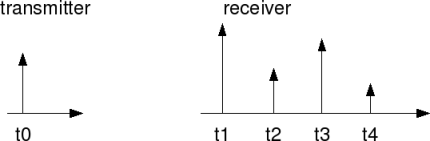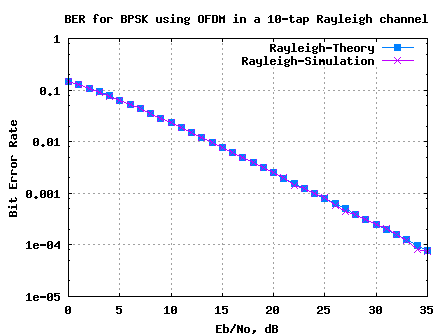- DSP log - http://www.dsplog.com -

BER for BPSK in OFDM with Rayleigh multipath channel

Posted By Krishna Sankar On August 26, 2008 @ 5:54 am In Channel,OFDM | 319 Comments

Mr. Lealem Tamirat, in a comment on BER for BPSK in Rayleigh channel , wondered about the performance of an OFDM modulated system in a frequency selective Rayeligh fading channel. My response was that,

Though the total channel is a frequency selective channel, the channel experienced by each subcarrier in an OFDM system is a flat fading channel with each subcarrier experiencing independent Rayleigh fading.

So, assuming that the number of taps in the channel is lower than the cyclic prefix duration (which ensures that there is no inter symbol interference), the BER for BPSK with OFDM in a Rayleigh fading channel should be same as the result obtained for BER for BPSK in Rayleigh fading channel.

Let us try to define a quick simulation to confirm the claim.

## OFDM system

Let us use an OFDM system loosely based on IEEE 802.11a specifications.

 Parameter Value FFT size. nFFT 64 Number of used subcarriers. nDSC 52 FFT Sampling frequency 20MHz Subcarrier spacing 312.5kHz Used subcarrier index {-26 to -1, +1 to +26} Cylcic prefix duration, Tcp 0.8us Data symbol duration, Td 3.2us Total Symbol duration, Ts 4us

You may refer to post Understanding an OFDM Transmission  and the post BPSK BER with OFDM modulation  for getting a better understanding of the above mentioned parameters.

## Eb/No and Es/No in OFDM

The relation between symbol energy and the bit energy is as follows:
.

Expressing in decibels,

.

## Rayleigh multipath channel model

As defined in the post on Rayleigh multipath channel model , the channel was modelled as n-tap channel with each the real and imaginary part of each tap being an independent Gaussian random variable. The impulse response is,

,

where
is the channel coefficient of the 1st tap,

is the channel coefficient of the 2nd tap and so on.

The real and imaginary part of each tap is an independent Gaussian random variable with mean 0 and variance 1/2.

The term is for normalizing the average channel power over multiple channel realizations to 1.Figure: Impulse response of a multipath channel

## Cyclic prefix

In the post on Cyclic Prefix in OFDM , we discussed the need for cyclic prefix and how it plays the role of a buffer region where delayed information from the previous symbols can get stored. Further, since addition of sinusoidal with a delayed version of the sinusoidal does not change the frequency of the sinusoidal (affects only the amplitude and phase), the orthogonality across subcarriers is not lost even in presence of multipath.

Since the defined cyclic prefix duration is 0.8us duration (16 samples at 20MHz), the Rayleigh channel is chosen to be of duration 0.5us (10 taps).

## Expected Bit Error Rate

From the post on BER for BPSK in Rayleigh channel, the BER for BPSK in a Rayleigh fading channel is defined as

.

I recall reading that Fourier transform of a Gaussian random variable is still has a Gaussian distribution. So, I am expecting that the frequency response of a complex Gaussian random variable (a.k.a Rayleigh fading channel) will be still be independent complex Gaussian random variable over all the frequencies.

Note:
I will update the post, once I am able to locate the proof for “frequency response of a complex Gaussian random variable is also complex Gaussian (and is independent with frequency)“.

Given so, the bit error error probability which we have derived for BER for BPSK in Rayleigh channel  holds good even in the case of OFDM.

## Simulation model

The attached Matlab/Octave simulation script performs the following:

(a) Generation of random binary sequence

(b) BPSK modulation i.e bit 0 represented as -1 and bit 1 represented as +1

(c) Assigning to multiple OFDM symbols where data subcarriers from -26 to -1 and +1 to +26 are used, adding cyclic prefix,

(d) Convolving each OFDM symbol with a 10-tap Rayleigh fading channel. The fading on each symbol is independent. The frequency response of fading channel on each symbol is computed and stored.

(e) Concatenation of multiple symbols to form a long transmit sequence

(g) Grouping the received vector into multiple symbols, removing cyclic prefix

(h) Converting the time domain received symbol into frequency domain

(i) Dividing the received symbol with the known frequency response of the channel

(j) Taking the desired subcarriers

(k) Demodulation and conversion to bits

(l) Counting the number of bit errors

(m) Repeating for multiple values of Eb/No

The simulation results are as shown in the plot below.Figure: BER plot for BPSK with OFDM modulation in a 10-tap Rayleigh fading channel

## Summary

1. The simulated BER results are in good agreement with the theoretical BER results.

2. Need to find the proof for frequency response of a complex Gaussian random variable is also complex Gaussian (and is independent with frequency).

Hope this helps. Happy learning.

URL to article: http://www.dsplog.com/2008/08/26/ofdm-rayleigh-channel-ber-bpsk/

URLs in this post:

 BER for BPSK in Rayleigh channel: http://www.dsplog.com/2008/08/10/ber-bpsk-rayleigh-channel/#comment-1155

 Understanding an OFDM Transmission: http://www.dsplog.com/2008/02/03/understanding-an-ofdm-transmission/

 BPSK BER with OFDM modulation: http://www.dsplog.com/2008/06/10/ofdm-bpsk-bit-error/

 Rayleigh multipath channel model: http://www.dsplog.com/2008/07/14/rayleigh-multipath-channel/

 Cyclic Prefix in OFDM: http://www.dsplog.com/2008/02/17/cylcic-prefix-in-orthogonal-frequency-division-multiplexing/

 BER for BPSK in Rayleigh channel, : http://www.dsplog.com/2008/08/10/ber-bpsk-rayleigh-channel/

 Matlab/Octave script for BER simulation of BPSK in a 10-tap Rayleigh fading channel: http://www.dsplog.com/db-install/wp-content/uploads/2008/08/script_ber_bpsk_ofdm_rayleigh_channel.m